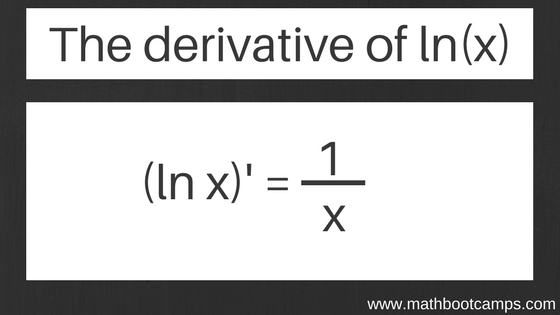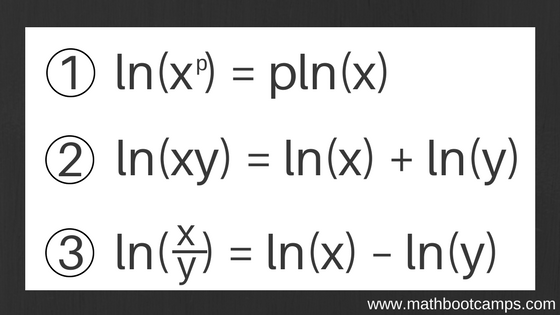﻿ The derivative of lnx and examples - MathBootCamps

# The derivative of lnx and examples

Part of calculus is memorizing the basic derivative rules like the product rule, the power rule, or the chain rule. One of the rules you will see come up often is the rule for the derivative of lnx. In the following lesson, we will look at some examples of how to apply this rule to finding different types of derivatives. We will also see how using the laws of logarithms can help make taking these kinds of derivatives even easier.

## Examples of finding the derivative of lnxRemember that when taking the derivative, you can break the derivative up over addition/subtraction, and you can take out constants. This allows us to find the following.

### Example

$$\left(3\ln(x)\right)^{\prime} = 3\left(\dfrac{1}{x}\right) = \dfrac{3}{x}$$

### Example

$$\left(\dfrac{\ln(x)}{5}\right)^{\prime} = \dfrac{1}{5}\left(\ln(x)\right)^{\prime} = \left(\dfrac{1}{5}\right)\left(\dfrac{1}{x}\right) = \dfrac{1}{5x}$$

### Example

$$\left(2x^2 – \ln(x)\right)^{\prime} = 4x – \dfrac{1}{x}$$

These show you the more straightforward types of derivatives you can find using this rule. But, if we combine this with the laws of logarithms we can do even more.

## Using the laws of logarithms to help

For some derivatives involving ln(x), you will find that the laws of logarithms are helpful. In terms of ln(x), these state:Using these, you can expand an expression before trying to find the derivative, as you can see in the next few examples. Here, we will do into a little more detail than with the examples above.

### Example

Find the derivative of the function:
$$y = \ln(x^2)$$

### Solution

Before applying any calculus rules, first expand the expression using the laws of logarithms. Here, we can use rule (1). This step is all algebra; no calculus is done until after we expand the expression.

$$y = \ln(x^2) = 2\ln(x)$$

Now, take the derivative. This is the calculus step.

\begin{align} y^{\prime} &= \left(2\ln(x)\right)^{\prime}\\ &= 2\left(\ln(x)\right)^{\prime}\\ &= 2\left(\dfrac{1}{x}\right)\\ &= \boxed{\dfrac{2}{x}}\end{align}

In the example above, only one rule was needed to fully expand the expression. The next example shows you how to apply more than one rule.

### Example

Find the derivative of the function.
$$y = \ln(5x^4)$$

Before taking the derivative, we will expand this expression. Since the exponent is only on the x, we will need to first break this up as a product, using rule (2) above. Then, we can apply rule (1).

$$y = \ln(5x^4) = \ln(5) + \ln(x^4) = \ln(5) + 4\ln(x)$$

Now take the derivative of the expanded form of the function, and then simplify.

\begin{align} y^{\prime} &= \left(\ln(5) + 4\ln(x)\right)^{\prime}\\ &= \left(\ln(5)\right)^{\prime} + 4\left(\ln(x)\right)^{\prime}\\ &= 4\left(\dfrac{1}{x}\right)\\ &= \boxed{\dfrac{4}{x}}\end{align}

You may be wondering what happened to $$\ln(5)$$. Remember – this is a constant. If you check your calculator, you will find that $$\ln(5) \approx 1.61$$. The derivative of a constant is zero.

None of these examples have used rule (3), so let’s look at one more example to see how that might be applied.

### Example

Find the derivative of the function.
$$y = \ln\left(\dfrac{6}{x^2}\right)$$

Here we have a fraction, which we can expand with rule (3), and then a power, which we can expand with rule (1). Remember that this is just algebra – no calculus is involved just yet.

$$y = \ln\left(\dfrac{6}{x^2}\right) = \ln(6) – \ln(x^2) = \ln(6) – 2\ln(x)$$

Now that we have $$\ln(x)$$ by itself, we can apply the derivative rule for the natural log.

\begin{align}y^{\prime} &= \left(\ln(6) – 2\ln(x)\right)^{\prime}\\ &= \left(\ln(6)\right)^{\prime} – 2\left(\ln(x)\right)^{\prime}\\ &= -2\left(\dfrac{1}{x}\right)\\ &= \boxed{-\dfrac{2}{x}}\end{align}

As in the previous example, $$\ln(6)$$ is a constant, so its derivative is zero.

## Combining with other rules

Each of the derivatives above could also have been found using the chain rule. As you study calculus, you will find that many problems have multiple possible approaches. However, there are some cases where you have no choice. For example, consider the following function.

$$y = \ln(3x^2 + 5)$$

Since this is not simply $$\ln(x)$$, we cannot apply the basic rule for the derivative of the natural log. Also, since there is no rule about breaking up a logarithm over addition (you can’t just break this into two parts), we can’t expand the expression like we did above. Instead, here, you MUST use the chain rule. Let’s see how that would work.

### Example

Find the derivative of the function.
$$y = \ln(3x^2 + 5)$$

Apply the chain rule.

\begin{align}y^{\prime} &= \dfrac{1}{3x^2 + 5}\left(3x^2 + 5\right)^{\prime}\\ y^{\prime} &= \dfrac{1}{3x^2 + 5}\left(6x\right)\end{align}

Since this cannot be simplified, we have our final answer.

\begin{align}y^{\prime} &= \dfrac{1}{3x^2 + 5}\left(6x\right)\\ &= \boxed{\dfrac{6x}{3x^2+5}}\end{align}

In some problems, you will find that there is a bit of algebra in the last step, with common factors cancelling. Be sure to always check for this.

## Summary

Remember the following points when finding the derivative of ln(x):

• The derivative of $$\ln(x)$$ is $$\dfrac{1}{x}$$.
• In certain situations, you can apply the laws of logarithms to the function first, and then take the derivative.
• Values like $$\ln(5)$$ and $$\ln(2)$$ are constants; their derivatives are zero.
• $$\ln(x + y)$$ DOES NOT EQUAL $$\ln(x) + \ln(y)$$; for a function with addition inside the natural log, you need the chain rule.
• $$\ln(x – y)$$ DOES NOT EQUAL $$\ln(x) – \ln(y)$$; for a function with subtraction inside the natural log, you need the chain rule.